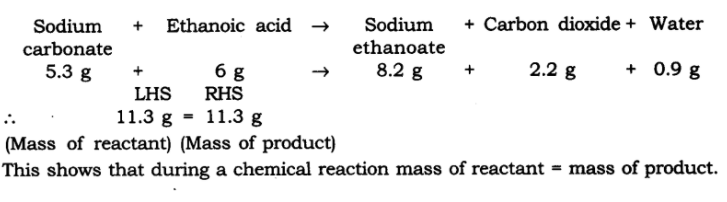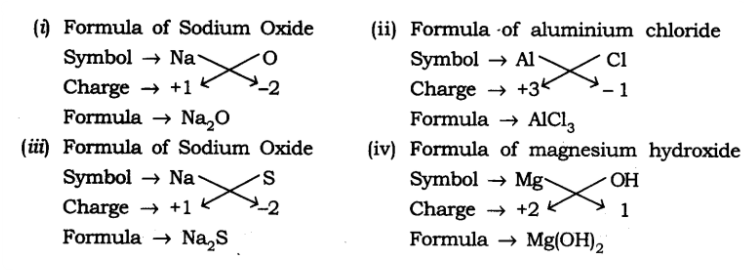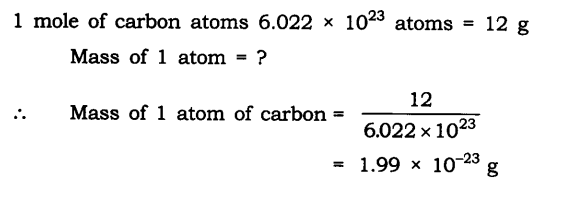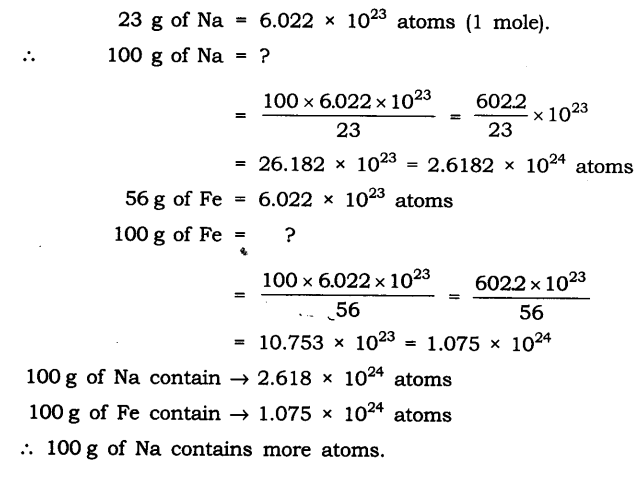# NCERT Solutions for Class 9 Science Chapter 3 Atoms And Molecules

Class 9 Science NCERT Textbook Page 32

Question:- 1. In a reaction, 5.3 g of sodium carbonate reacted with 6 g of acetic acid. The products were 2.2 g of carbon dioxide, 0.9 g water and 8.2 g of sodium Q acetate. Show that these observations are in agreement with the law of conservation of mass.

sodium carbonate + acetic acid → sodium acetate + carbon dioxide + waterQuestion:- 2. Hydrogen and oxygen combine in the ratio of 1:8 by mass to form water. What mass of oxygen gas would be required to react completely with 3 g of hydrogen gas?

Answer:- Ratio of H : O by mass in water is:

Hydrogen : Oxygen —> H2O

1 : 8 = 3 : x

x = 8 x 3

x = 24 g

Therefore 24 g of oxygen gas would be required to react completely with 3 g of hydrogen gas.

Question:- 3. Which postulate of Dalton’s atomic theory is the result of the law of conservation of mass?

Answer:- “Atoms are indivisible particles, which cannot be created or destroyed in a chemical reaction”.

Question:- 4. Which postulate of Dalton’s atomic theory can explain the law of definite proportions?

Answer:- “The relative number and kinds of atoms are constant in a given compound”.

Class 9 Science NCERT Textbook Page 35

Question:- 1. Define the atomic mass unit.

Answer:-  An atomic mass unit is a unit of mass used to express atomic and molecular weights, equal to one twelfth of the mass of an atom of carbon-12.

Question:- 2. Why is it not possible to see an atom with naked eyes?

Answer:-  Atoms are too small to be seen with naked eyes. Size of an atom is measured in nanometres. Hence, it is not possible to see an atom with naked eyes.

Class 9 Science NCERT Textbook Page 39

Question:- 1. Write down the formulae of

(i) sodium oxide

(ii) aluminium chloride

(iii) sodium suphide

(iv) magnesium hydroxideQuestion:- 2. Write down the names of Q compounds represented by the following formulae:-

(i) Al2(SO4)3

(ii) CaCl2

(iii) K2SO4

(iv) KNO3

(v) CaCO3

(i) Al2(SO4)3   :-  Aluminium sulphate

(ii) CaCl2           :- Calcium chloride

(iii) K2SO4        :-  Potassium sulphate

(iv) KNO3         :-  Potassium nitrate

(v) CaCO3      :-  Calcium carbonate

Question:- 3. What is meant by the term chemical formula?

Answer:- The chemical formula of the compound is a symbolic representation of its composition. For example:- The chemical formula of hydrochloric acid is HCl.

Question:- 4. How many atoms are present in a

(i) H2S molecule and

(ii) PO4 3– ion?

Answer:- (i) Total 3 atoms (2 atoms of hydrogen and 1 atom of sulphur ) are present in H2S molecule

(ii) Total 5 atoms (1 atom of phosphorus and 4 atoms of oxygen) are present in PO4 3–  ion.

Class 9 Science NCERT Textbook Page 40

Question:- 1. Calculate the molecular masses of H2 , O2 , Cl2, CO2, CH4, C2H6, C2H4, NH3, CH3OH.

The molecular mass of H2 = 2 x atomic mass of H = 2 x 1u = 2u

The molecular mass of O2 = 2 x atomic mass of O = 2 x 16u = 32u

The molecular mass of Cl2  =  2 x = atomic mass of Cl = 2 x 35.5u = 71u

The molecular mass of CO2  =  atomic mass of C + 2 x atomic mass of O = 12 + 2×16u = 44u

The molecular mass of CH4 =  atomic mass of C + 4 x atomic mass of H = 12 +  4 x 1 u = 16u

The molecular mass of C2H6 =  2 x atomic mass of C + 6 x atomic mass of H = 2 x 12 + 6 x 1 u=24+6=30u

The molecular mass of C2H4 = 2 x atomic mass of C + 4 x atomic mass of H = 2x 12 + 4 x 1 u=24+4=28u

The molecular mass of NH3 = atomic mass of N + 3 x atomic mass of H = 14 +3 x 1 u= 17u

The molecular mass of CH3OH  =  atomic mass of C + 3x atomic mass of H + atomic mass of O + atomic mass of H = 12 + 3×1+16+1 u= 12+3+17 u = 32u

Question:- 2. Calculate the formula unit masses of ZnO, Na2O, K2CO3, given atomic masses of Zn = 65u, (Na = 23 u,  K=39u, C = 12u, and O=16u.)

Answer:-  The formula unit mass of ZnO

= Atomic mass of Zn + Atomic mass of O

= 65u + 16u = 81u

The formula unit mass of Na2O

= 2 x Atomic mass of Na + Atomic mass of O

= (2 x 23)u + 16u

= 46u + 16u = 62u

The formula unit mass of K2CO3

= 2 x Atomic mass of K + Atomic mass of C + 3 x Atomic mass of O

= (2 x 39)u + 12u + (3 x 16)u

= 78u + 12u + 48u = 138u

Class 9 Science NCERT Textbook Page 42

Question:- 1. If one mole of carbon atoms weighs 12 grams, what is the mass (in grams) of 1 atom of carbon?Question:- 2. Which has more number of atoms, 100 grams of sodium or 100 grams of iron (given, atomic Q mass of Na = 23 u, Fe = 56 u)?## Exercises

Question:- 1. A 0.24 g sample of compound of oxygen and boron was found by analysis to contain 0.096 g of boron and 0.144 g of oxygen. Calculate the percentage composition of the compound by weight.

Answer:- Given:- Total mass of Compound = 0.24 g

Mass of boron = 0.096 g

Mass of oxygen = 0.144 g

Thus, percentage of boron by weight in the compound = 0.096 / 0.24 × 100% = 40%

And, percentage of oxygen by weight in the compound = 0.144 / 0.24 × 100% = 60%

Question:- 2. When 3.0 g of carbon is burnt in 8.00 g oxygen, 11.00 g of carbon dioxide is produced. What mass of carbon dioxide will be formed when 3.00 g of carbon is burnt in 50.00 g of oxygen? Which law of chemical combination will govern your answer?

Answer:- It is said that 3.0 g of carbon combines with 8.0 g of oxygen to give 11.0 of carbon dioxide.

If we have 3 g of carbon to burn in 50 g of oxygen, then 3 g of carbon will react with only 8 g of oxygen. The remaining 50-8 = 42 g of oxygen will be left un-reactive.

Then also in this case, only 11 g of carbon dioxide will be formed.

The law of constant proportions will govern our answer.

Question:- 3. What are polyatomic ions? Give examples.

Answer:-  A group of atoms carrying a charge either + or – is known as a polyatomic ion.  For example:- OH, SO42- , CO32- .

Question:- 4. Write the chemical formulae of the following.

(a) Magnesium chloride

(b) Calcium oxide

(c) Copper nitrate

(d) Aluminium chloride

(e) Calcium carbonate.

Answer:- (a) Magnesium chloride –  MgCl2

(b) Calcium oxide – CaO

(c) Copper nitrate – Cu(NO3)2

(d) Aluminium chloride – AlCl3

(e) Calcium carbonate – CaCO3

Question:- 5. Give the names of the elements present in the following  compounds.

(a) Quick lime

(b) Hydrogen bromide

(c) Baking powder

(d) Potassium sulphate.

Answer:-  (a) Quick lime – Calcium and oxygen (CaO)

(b) Hydrogen bromide – Hydrogen and bromine (HBr)

(c) Baking powder – Sodium, Carbon, Hydrogen, Oxygen (NaHCO3)

(d) Potassium sulphate – Sulphur, Oxygen, Potassium (K2SO4)

Question:- 6. Calculate the molar mass of the following substances.

(a) Ethyne, C2H2

(b) Sulphur molecule, S8

(c) Phosphorus molecule, P4 (Atomic mass of phosphorus = 31)

(d) Hydrochloric acid, HCl

(e) Nitric acid, HNO3

Answer:- (a) Molar mass of Ethyne = C2H2 = 2 x Mass of Carbon+2 x Mass of Hydrogen = 2×12 + 2×1 = 24+2 = 26g

(b) Molar mass of Sulphur molecule S8 = 8 x Mass of Sulphur = 8  x 32 = 256g

(c) Molar mass of  Phosphorus molecule, P4 = 4 x Mass of Phosphorus = 4 x 31 = 124g

(d) Molar mass of Hydrochloric acid, HCl = Mass of Hydrogen + Mass of Chloride = 1+35.5 = 36.5g

(e) Molar mass of Nitric acid, HNO3 = Mass of Hydrogen + Mass of Nitrogen + 3 x Mass of O xygen = 1 + 14+ 3×16 = 63g

Question:- 7. What is the mass of—

(a) 1 mole of nitrogen atoms?

(b) 4 moles of aluminium atoms (Atomic mass of aluminium = 27)?

(c) 10 moles of sodium sulphite (Na2SO3)?

Answer:- (a) The mass of 1 mole of nitrogen atom is 14 g.

(b) The mass of 4 moles of aluminium atom is (4 × 27) g = 108 g

(c) The mass of 10 moles of sodium sulphite (Na2SO3) is 10 × 2×23 + 32 + 3×16 g = 10×126 g = 1260 g

Question:- 8. Convert into mole.

(a) 12 g of oxygen gas

(b) 20 g of water

(c) 22 g of carbon dioxide.

Answer:- (a) Given: Mass of oxygen gas=12g

Molar mass of oxygen gas = 2 Mass of Oxygen =  2 x 16 = 32g

Number of moles = Mass given / molar mass of oxygen gas = 12/32 =  0.375 moles

(b) Given: Mass of water = 20g

Molar mass of water = 2 x Mass of Hydrogen + Mass of Oxygen = 2 x 1 + 16 = 18g

Number of moles = Mass given / molar mass of water

= 20/18 = 1.11 moles

(c) Given: Mass of carbon dioxide = 22g

Molar mass of carbon dioxide = Mass of C + 2 x Mass of Oxygen = 12 + 2x 16 = 12+32=44g

Number of moles = Mass given/ molar mass of carbon dioxide = 22/44 = 0.5 moles

Question:- 9. What is the mass of:

(a) 0.2 mole of oxygen atoms?

(b) 0.5 mole of water molecules?

Answer:- (a) Mass of 1 mole of oxygen atoms = 16u, hence it weighs 16g

Mass of 0.2 moles of oxygen atoms = 0.2 x 16 = 3.2g

(b) Mass of 1 mole of water molecules = 18u, hence it weighs 18g

Mass of 0.5 moles of water molecules = 0.5 x 18 = 9g

Question:- 10. Calculate the number of molecules of sulphur (S8 ) present in 16 g of solid sulphur.

Answer:- Molecular mass of Sulphur (S8) = 8x Mass of Sulphur = 8×32 = 256g

Mass given = 16g

Number of moles = mass given/ molar mass of sulphur

= 16/256 = 0.0625 moles

To calculate the number of molecules of sulphur in 16g of solid sulphur:

Number of molecules = Number of moles x Avogadro number

= 0.0625 x 6.022 x 10²³ molecules

= 3.763 x 1022 molecules

Question:- 11. Calculate the number of aluminium ions present in 0.051 g of aluminium oxide. (Hint: The mass of an ion is the same as that of an atom of the same element. Atomic mass of Al = 27 u)

Answer:- Mole of aluminium oxide (Al2O3) = 2×27 + 3×16 = 102 g

i.e., 102 g of Al2O3= 6.022 × 1023 molecules of Al2O3

Then, 0.051 g of Al2O3 contains = 6.022 × 1023 / 102 × 0.051 molecules

= 3.011×1020 molecules of Al2O3

The number of aluminium ions (Al3+) present in one molecule of aluminium oxide is 2.

Therefore, the number of aluminium ions (Al3+) present in 3.011 × 1020molecules (0.051 g ) of aluminium oxide (Al2O3) = 2 × 3.011 × 1020

= 6.022 × 1020